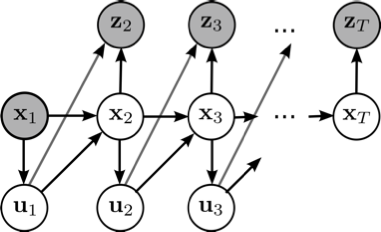# Probabilistic Machine Learning (RO5101 T)Univ.-Prof. Dr. Elmar Rueckert was teaching this course at the University of Luebeck in the winter semester in the years 2019 and 2020.

Teaching Assistant:
Nils Rottmann, M.Sc.

Language:
English only

## Course Details

Some remarks on the UzL Module idea: The lecture Probabilistic Machine Learning belongs to the Module Robot Learning (RO4100). In the winter semester, Prof. Dr. Elmar Rueckert is teaching the course Probabilistic Machine Learning (RO5101 T). In the summer semester, Prof. Dr. Elmar Rueckert is teaching the course Reinforcement Learning (RO5102 T). Important: Due to the study regulations, students have to attend both lectures to receive a final grade. Thus, there will be only a single written exam for both lectures. You can register for the written exam at the end of a semester.

## Important dates

• Written exam: 04. February 2021 (2nd appointment 04.03.2021)
• Assignment I: Freitag, 11. Dezember 2020, 23:00
• Assignment II: Freitag, 22. Januar 2021, 23:00

## The course topics are

1. Introduction to Probability Theory (Statistics refresher, Bayes Theorem, Common Probability distributions, Gaussian Calculus).
2. Linear Probabilistic Regression (Linear models, Maximum Likelihood, Bayes & Logistic Regression).
3. Nonlinear Probabilistic Regression (Radial basis function networks, Gaussian Processes, Recent research results in Robotic Movement Primitives, Hierarchical Bayesian & Mixture Models).
4. Probabilistic Inference for Filtering, Smoothing and Planning (Classic, Extended & Unscented Kalman Filters, Particle Filters, Gibbs Sampling, Recent research results in Neural Planning).
5. Probabilistic Optimization (Stochastic black-box Optimizer Covariance Matrix Analysis Evolutionary Strategies & Natural Evolutionary Strategies, Bayesian Optimization).

## The learning objectives / qualifications are

• Students get a comprehensive understanding of basic probability theory concepts and methods.
• Students learn to analyze the challenges in a task and to identify promising machine learning approaches.
• Students will understand the difference between deterministic and probabilistic algorithms and can define underlying assumptions and requirements.
• Students understand and can apply advanced regression, inference and optimization techniques to real world problems.
• Students know how to analyze the models’ results, improve the model parameters and can interpret the model predictions and their relevance.
• Students understand how the basic concepts are used in current state-of-the-art research in robot movement primitive learning and in neural planning.

## Interactive Online Lectures

In the lecture, Prof. Rueckert is using a self made lightboard to ensure an interactive and professional teaching environment. Have a look at the post on how to build such a lightboard. Here is an example recording.

## Requirements

Strong statistical and mathematical knowledge is required beforehand. It is highly recommended to attend the course Humanoid Robotics (RO5300) prior to attending this course. The students will also experiment with state-of-the-art machine learning methods and robotic simulation tools which require strong programming skills.